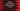# Python 3 program to count the number of blank spaces in a file## Python 3 program to count the number of blank spaces in a file :

In this tutorial, we will learn how to find the total number of blank spaces in a text file. This program will teach you basic file operations in python. You will learn how to iterate the lines of a text file and how to read each word and each character for each line.

You will also learn how to check if a character is a blank space or not. The program is easier than you think.

The following algorithm we are going to use in this example :

### Algorithm :

1. Store the file path in a variable. This is the path of the text file we are going to read. In this example, we are using one dummy _file path. Before running the program, _change this path to the file path you are going to test on your system. You can simply drag and drop one file on a terminal session to get the file path.
2. Create one variable to store the count of blank spaces and initialize it as ‘0’. We will keep updating this variable whenever we find any blank space in the file.
3. Open the file and read the lines one by one.
4. For each line, read all words one by one.
5. For each word, read all characters one by one. Actually, we are reading all the characters of the file one by one. But to do that, we need all these three steps.
6. Check for each character if it is a space or not. Python provides one inbuilt method to check if a character is a space or not. We will use that one.
7. If the character is a space, increment the count variable by one. The initial value of the count variable is 0. If any space is found, it will become 1, for the next space, it will be _2 _etc. At the end of the program, this variable will hold the total count of space in the file.
8. Finally, print out the value of _count _or the count of blank spaces to the user.

### Python 3 program :

``````file_path = "C:/new.txt"

space_count = 0

with open(file_path,'r') as f:
for line in f:
split_words = line.split()
for word in split_words:
for char in word:
if(char.isSpace):
space_count = space_count + 1

print("Total blank space found : ",space_count)``````